Divide Signed Integers

Divide Signed Integers

Every division problem has a related multiplication problem. Division and multiplication are opposite, or inverse, operations. Therefore, the rules for dividing integers are the same as multiplying integers.

Same signs = positive quotient

• The quotient of two numbers with the same signs is always positive.

Different signs = negative quotient

• The quotient of two numbers with different signs is always negative.

Examples.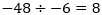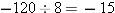Practice

1.    Divide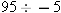2.    Simplify: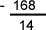3.    Divide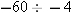4.    Find the quotient of -135 and 15.